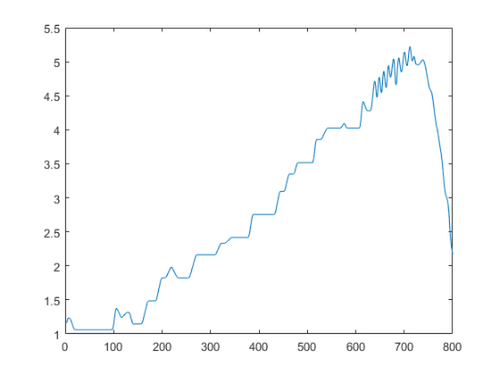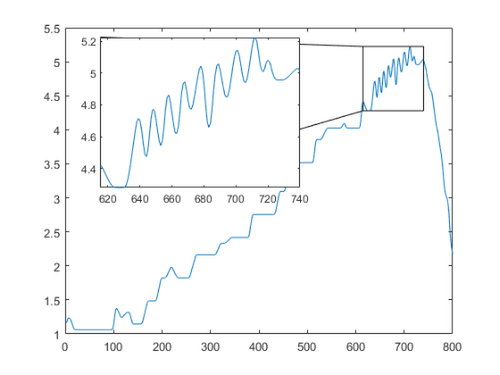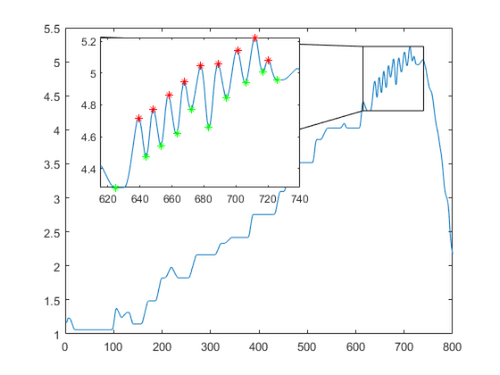# Zoom Plot

Sean's pick this week is zoomPlot by Kelsey Bower.

(No - it's not the matrix of faces that you see in a conference call!) My pick this week allows you to zoom in on specific region in a line plot.

Let's plot some data:

T = readtable('C:\Documents\MATLAB\FlightTestData.xlsx');
plot(T.LoadFactor);There are some interesting dynamics near the upper right hand corner. I'd like to show them with more detail. I can simply add a zoomPlot to this.

[~, z] = zoomPlot((1:800).', T.LoadFactor, [615 740], [0.2 0.5 0.4 0.4]);The detail is more clear. Now we can highlight the local minima and maxima in this zoomed in plot.

xzoom = 615:740;
hold(z, 'on');

plot(xzoom(imin),loadfactorzoom(imin), 'g*')Now if you did want a zoom plot like you'd see in a virtual meeting, use tiledlayout, which is new in R2019b and replaces subplot workflows.

figure
t = tiledlayout('flow', 'Padding', 'none', 'TileSpacing', 'none');
images = dir(fullfile(matlabroot, 'toolbox\images\imdata\*.jpg'));
for ii = 1:numel(images)
nexttile(t)
imshow(fullfile(images(ii).folder, images(ii).name))
end